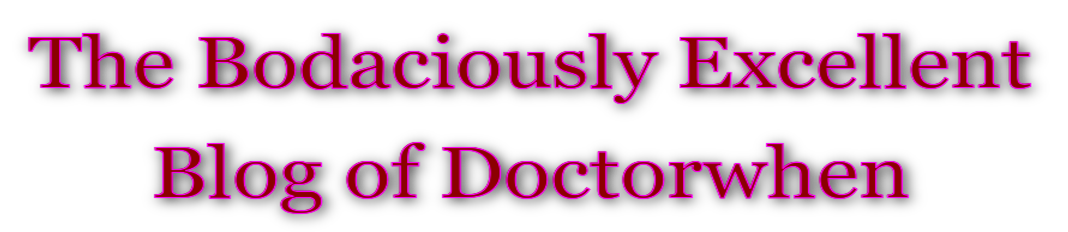# Mandelbox Zoom

## Mandelbox Zoom

An amazing zoom on the Mandelbox made with Mandelbulb 3D v1.53 and VirtualDub.

In mathematics, the mandelbox is a fractal with a boxlike shape found by Tom Lowe in 2010. It is defined in a similar way to the famous Mandelbrot set as the values of a parameter such that the origin does not escape to infinity under iteration of certain geometrical transformations. The mandelbox is defined as a map of continuous Julia sets, but, unlike the Mandelbrot set, can be defined in any number of dimensions.

The iteration applies to vector z as follows:

``````function iterate(z):
for each component in z:
if component > 1:
component := 2 - component
else if component < -1:
component := -2 - component

if magnitude of z < 0.5:
z := z * 4
else if magnitude of z < 1:
z := z / (magnitude of z)^2

z := scale * z + c``````

Here, c is the constant being tested, and scale is a real number.